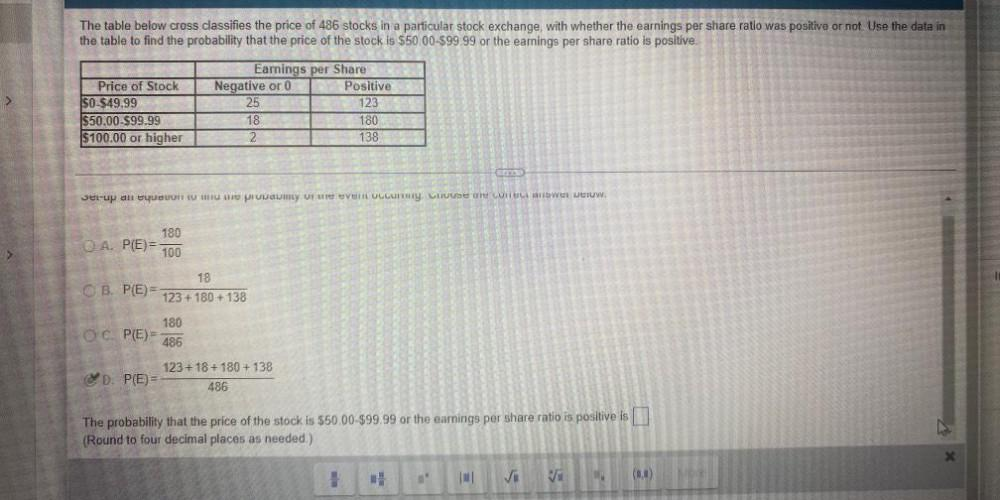Question:

# The table below cross classifies the price of 486 stocks in a particular stock exchange, with whether the earnings per share ratio was positive or not. Use the data in the table find the probability tThe table below cross classifies the price of 486 stocks in a particular stock exchange, with whether the earnings per share ratio was positive or not. Use the data in the table find the probability that the price of the stock is \$50.00-\$99.99 or the earnings per share ratio is positive. Earnings per Share Price of Stock Negative or 0 Positive \$0-\$49.99 25 123 \$50.00-\$99.99 18 180 \$100.00 or higher 2 138 A). P(E)=180/100 b)P(E) =18/(123+180 +138) C). P(E)= 180/486 D). P(E)=(123+18+180 +138)/486 The probability that the price of the stock is \$50.00-\$99.99 or the earnings per share ratio is positive is